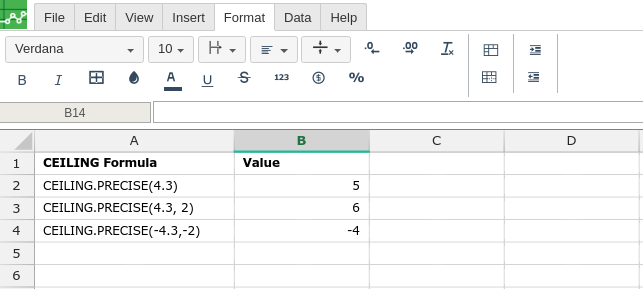# CEILING.PRECISE

### Definition

Returns a number that is rounded up to the nearest integer or to the nearest multiple of significance. Regardless of the sign of the number, the number is rounded up. However, if the number or the significance is zero, zero is returned.

### Syntax

CEILING.PRECISE(number, [significance])

The CEILING.PRECISE function syntax has the following arguments:

• Number    Required. The value to be rounded.

• Significance    Optional. The multiple to which number is to be rounded.

If significance is omitted, its default value is 1.

### Remarks

• The absolute value of the multiple is used, so that the CEILING.PRECISE function returns the mathematical ceiling irrespective of the signs of number and significance.

###Error.

User does not have sufficient privileges to access this Content